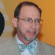cancel
Showing results for
Did you mean:New Contributor

## How can a subtance be more than 100% soluble in water?

For example, in the NIOSH guide to hazardous chemicals, Zinc Chloride Fume is 435% soluble in water at 70 degrees F.

Tags (1)Contributor III

## Re: How can a subtance be more than 100% soluble in water?

Dear Brian,

“Solubility” is really a relative term based on a ratio of (usually) a smaller amount of a solid or gas homogeneously mixed with a larger amount of liquid. We generally don’t call mixtures of liquids as some degree of solubility – although we do call them solutions.

In this case, it is just applying a convention of calling the solid added to water the solute and the water is the solvent. Many compounds can essentially absorb more water and retain a liquid solution state while becoming the major component. Zinc Chloride can form a solution (liquid) in water at a ratio of 435g ZnCl2 to 100g H2O. Thus, using the water as the basis for the “solvent” the “solubility” of ZnCl2 in H2O is 435/100 = 0.435 = 435%.

Aluminum nitrate and ammonium bromide are other examples of this phenomenon.

https://en.wikipedia.org/wiki/Solubility_table

Best regards,

Steven